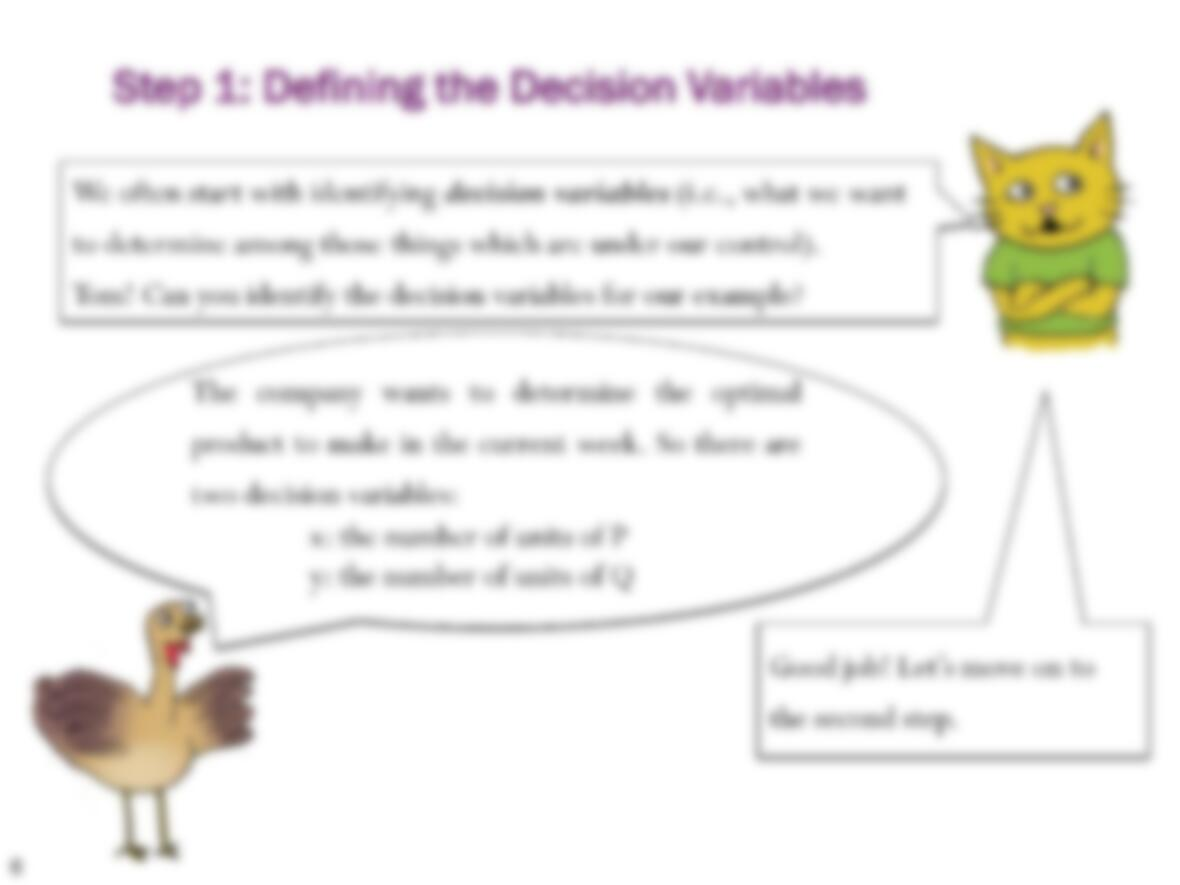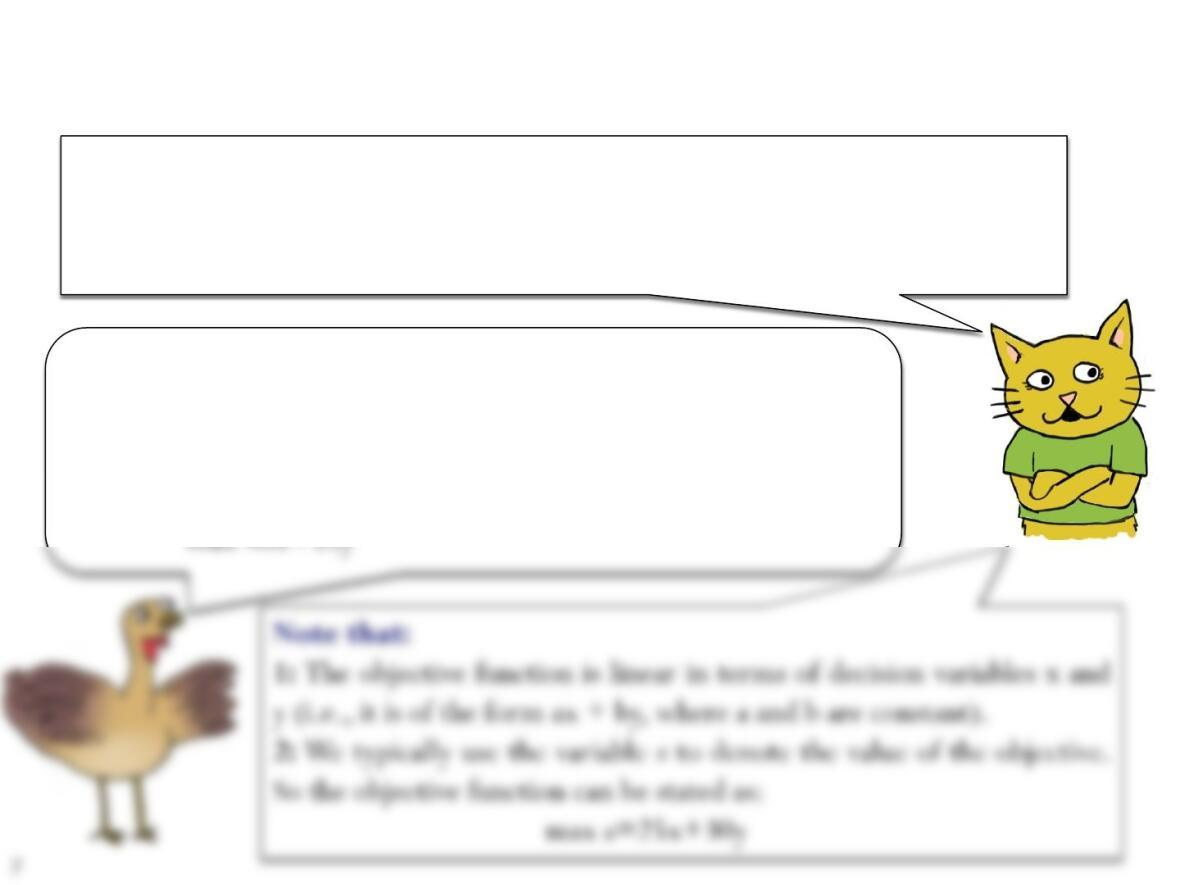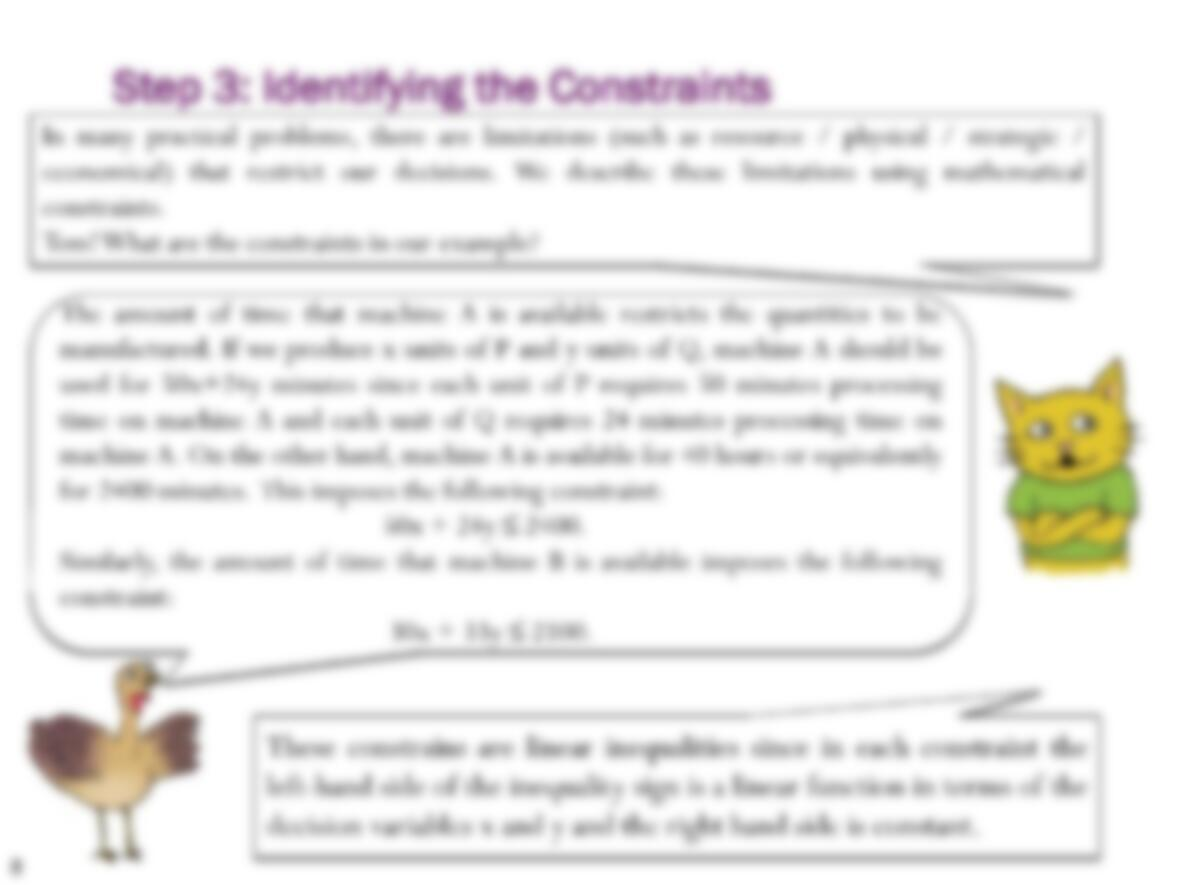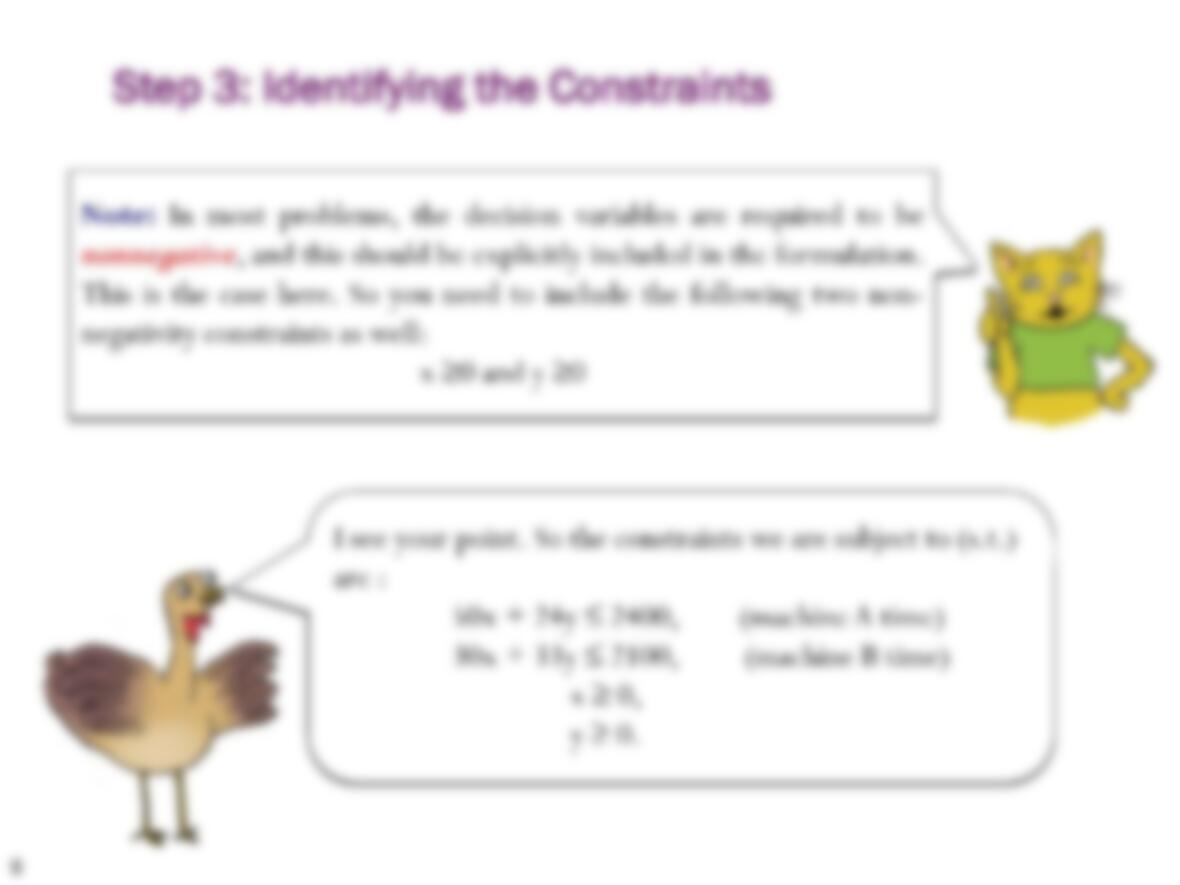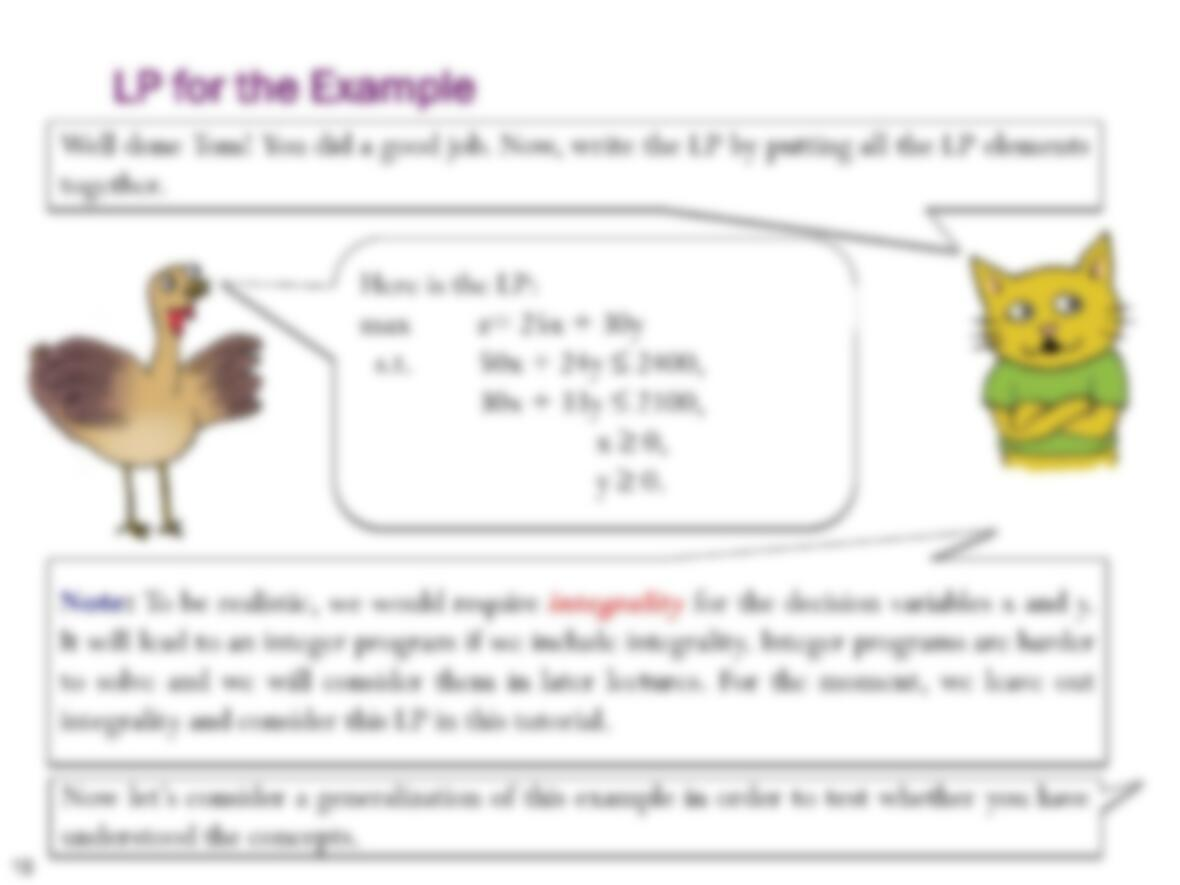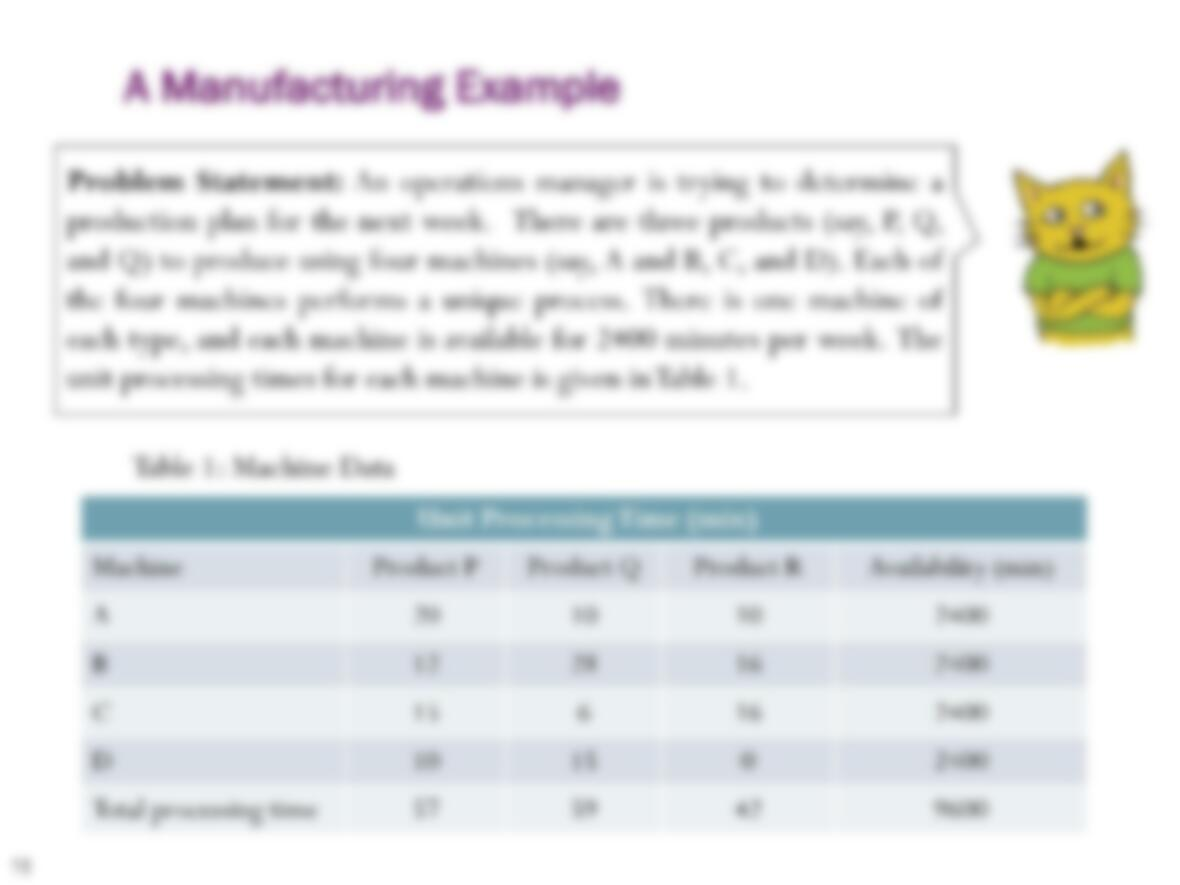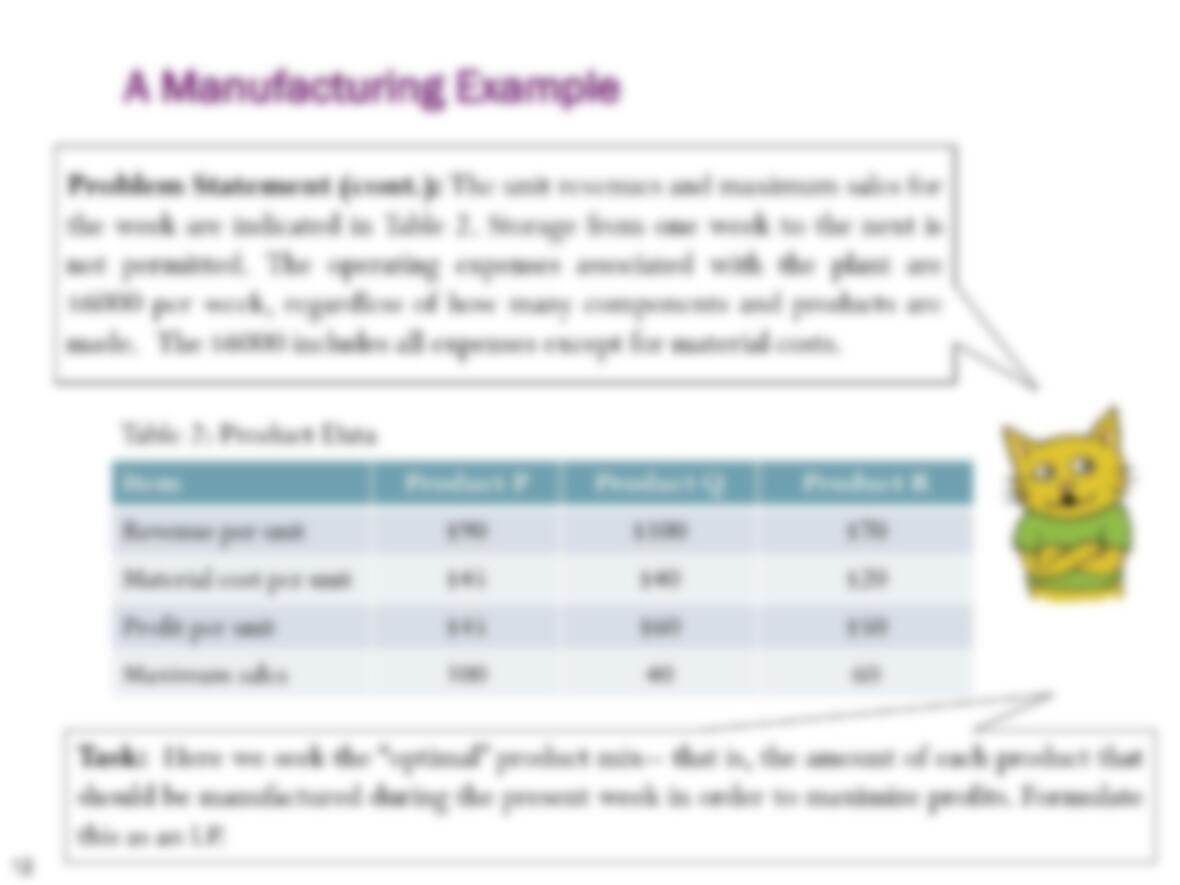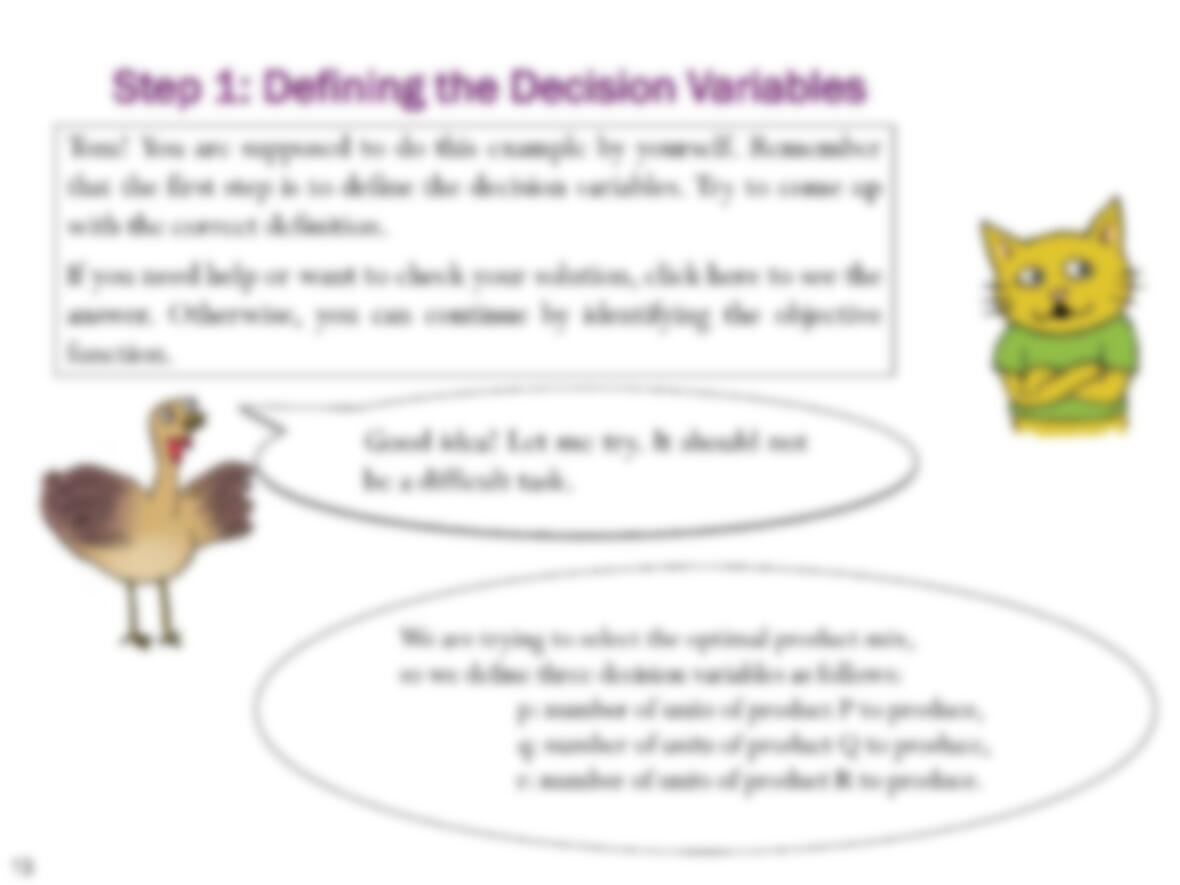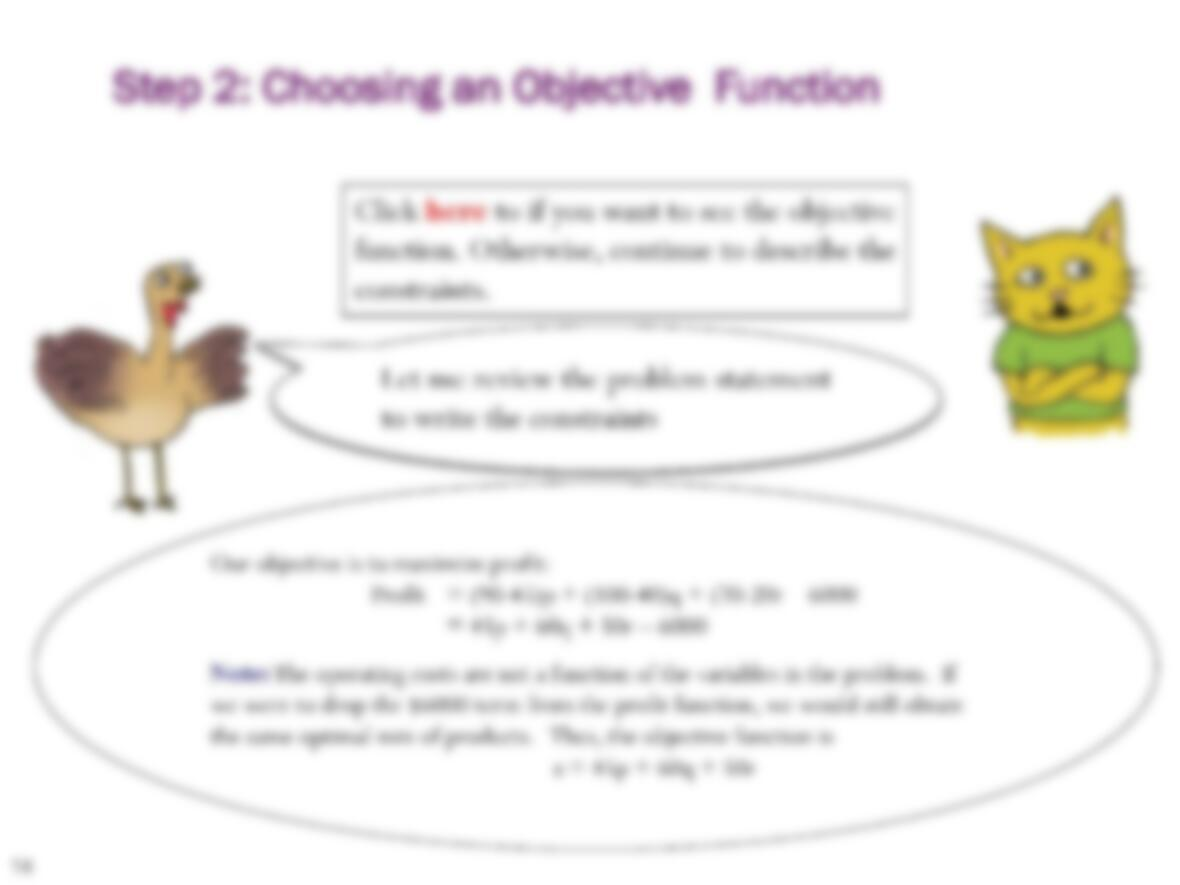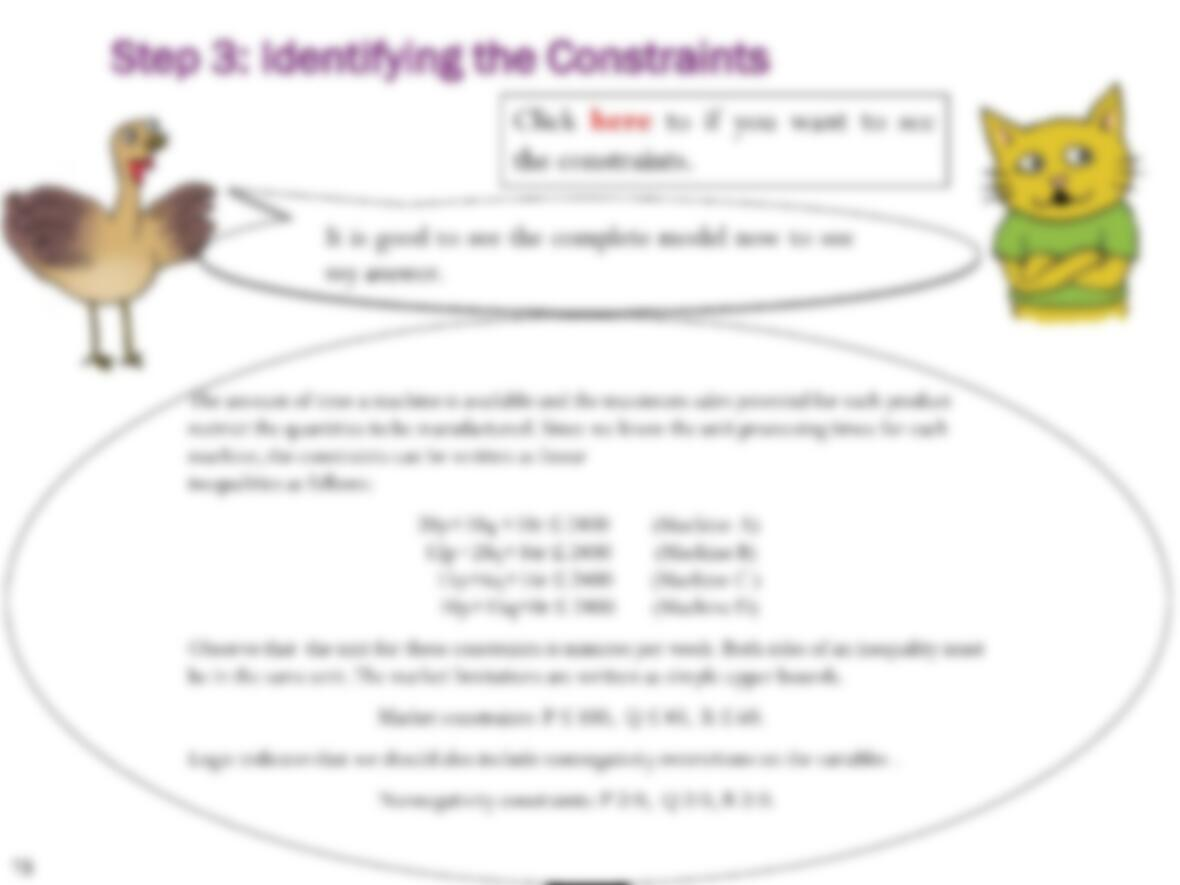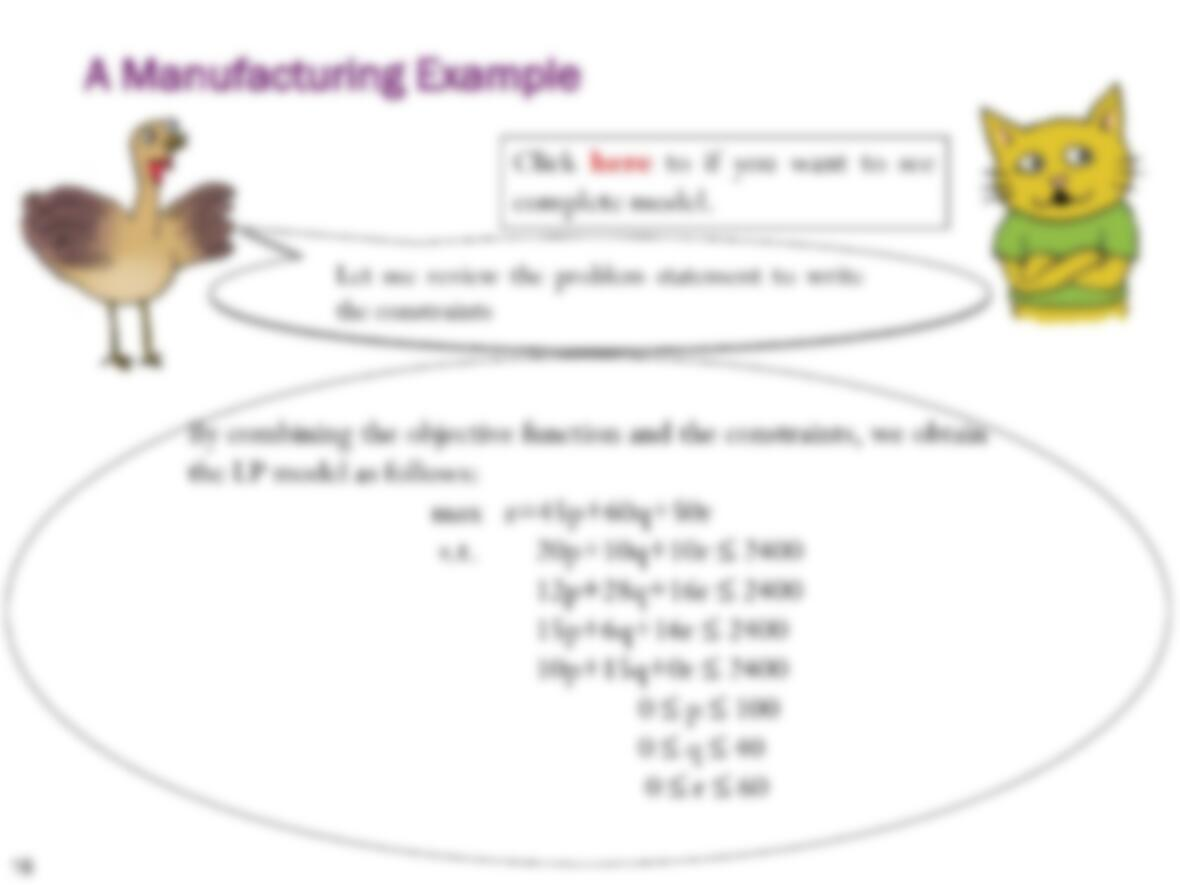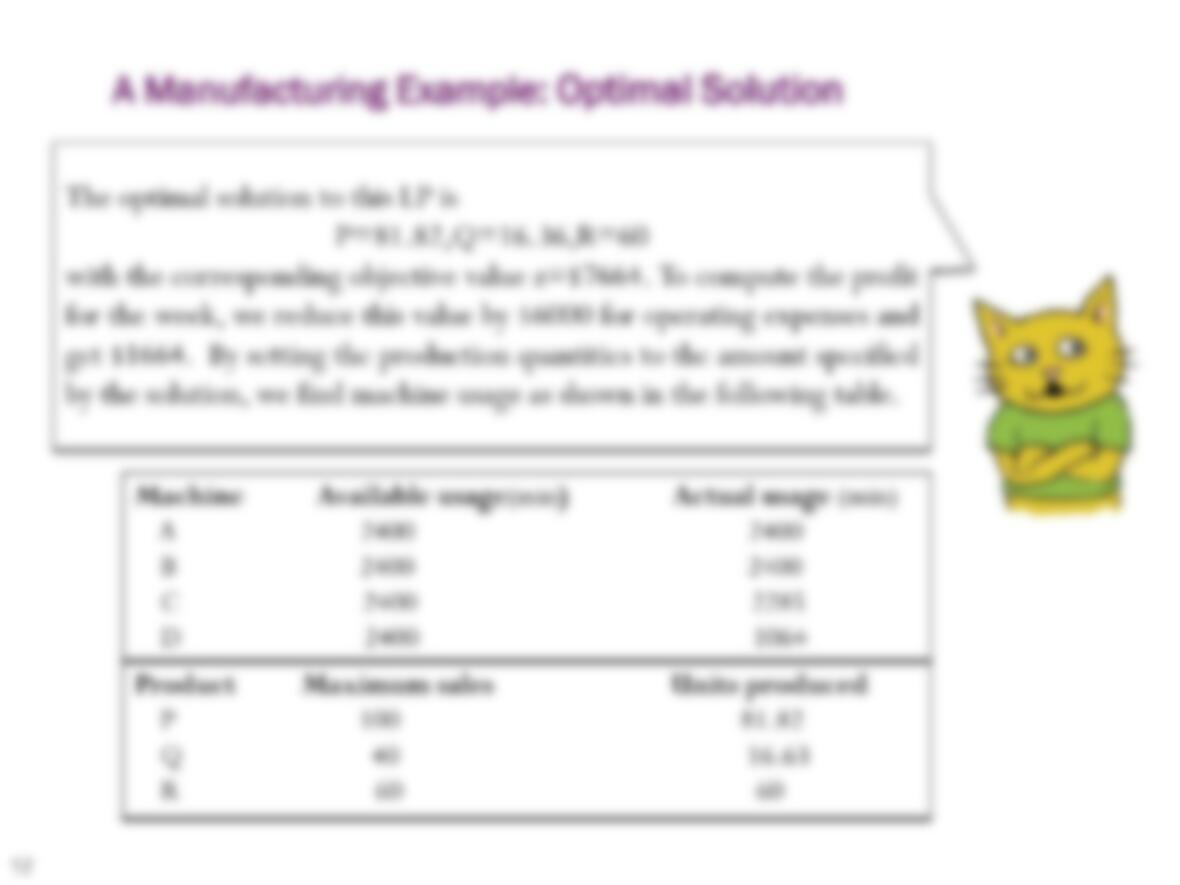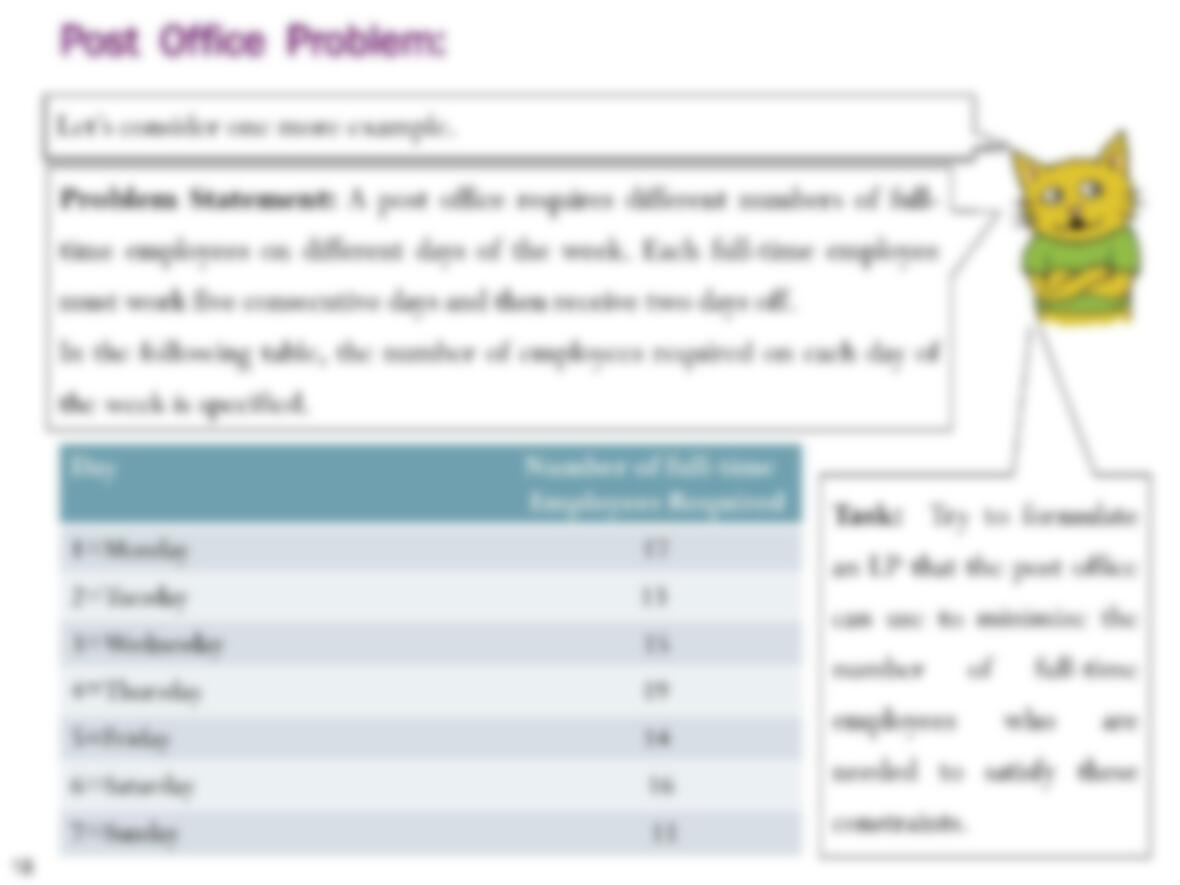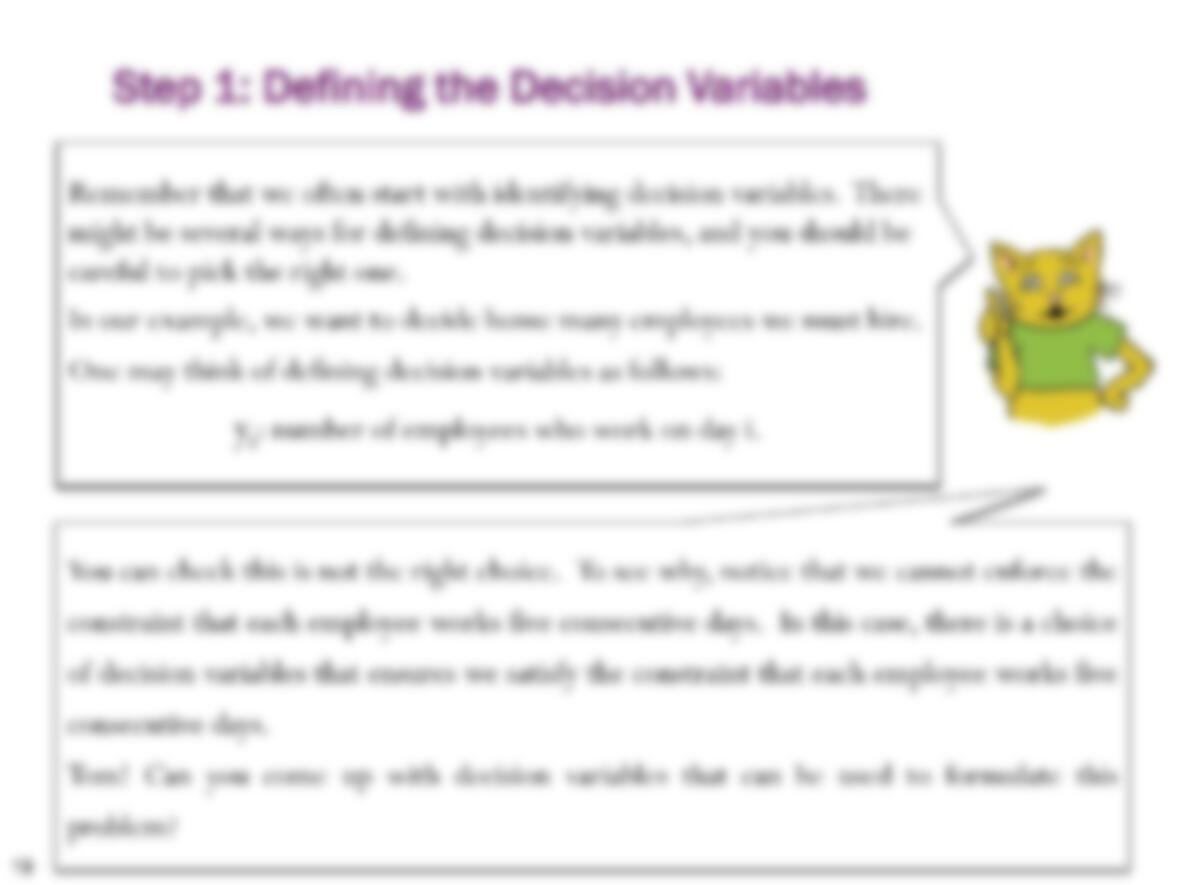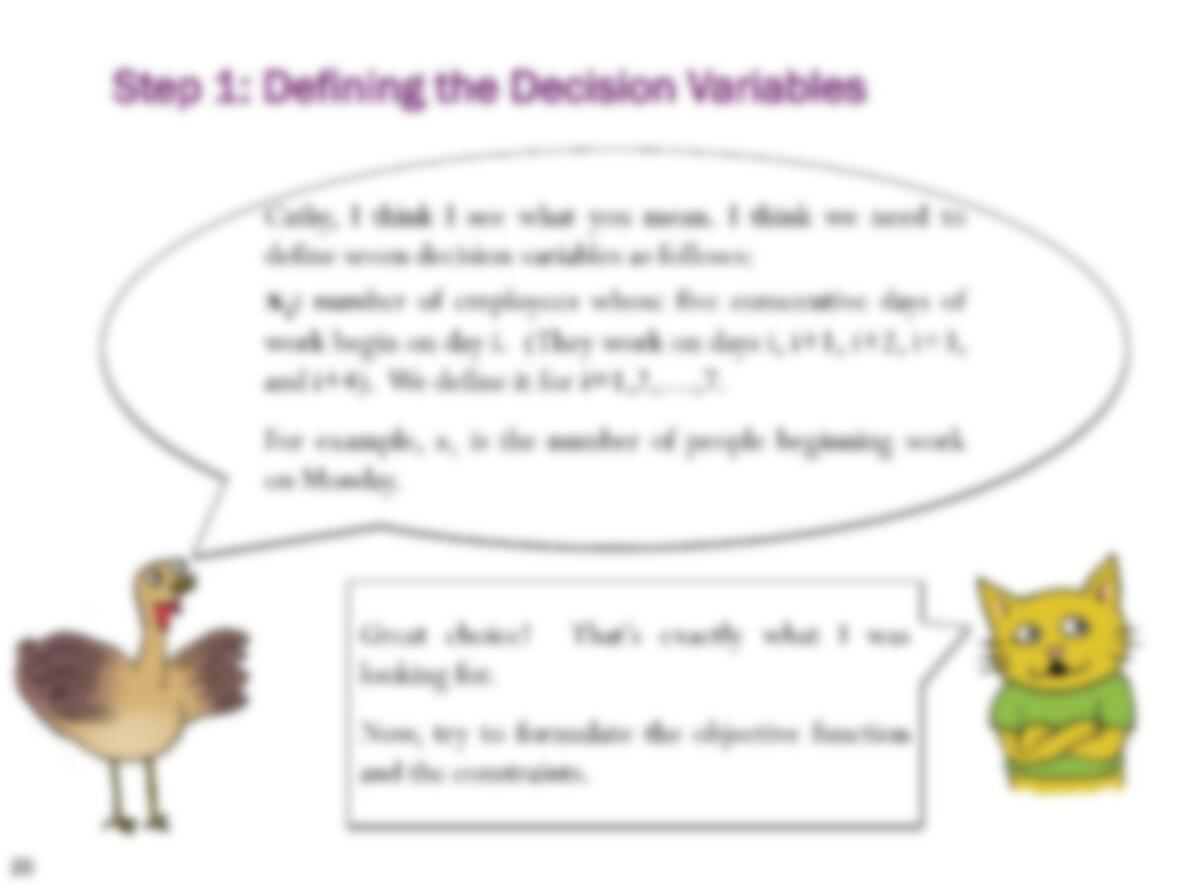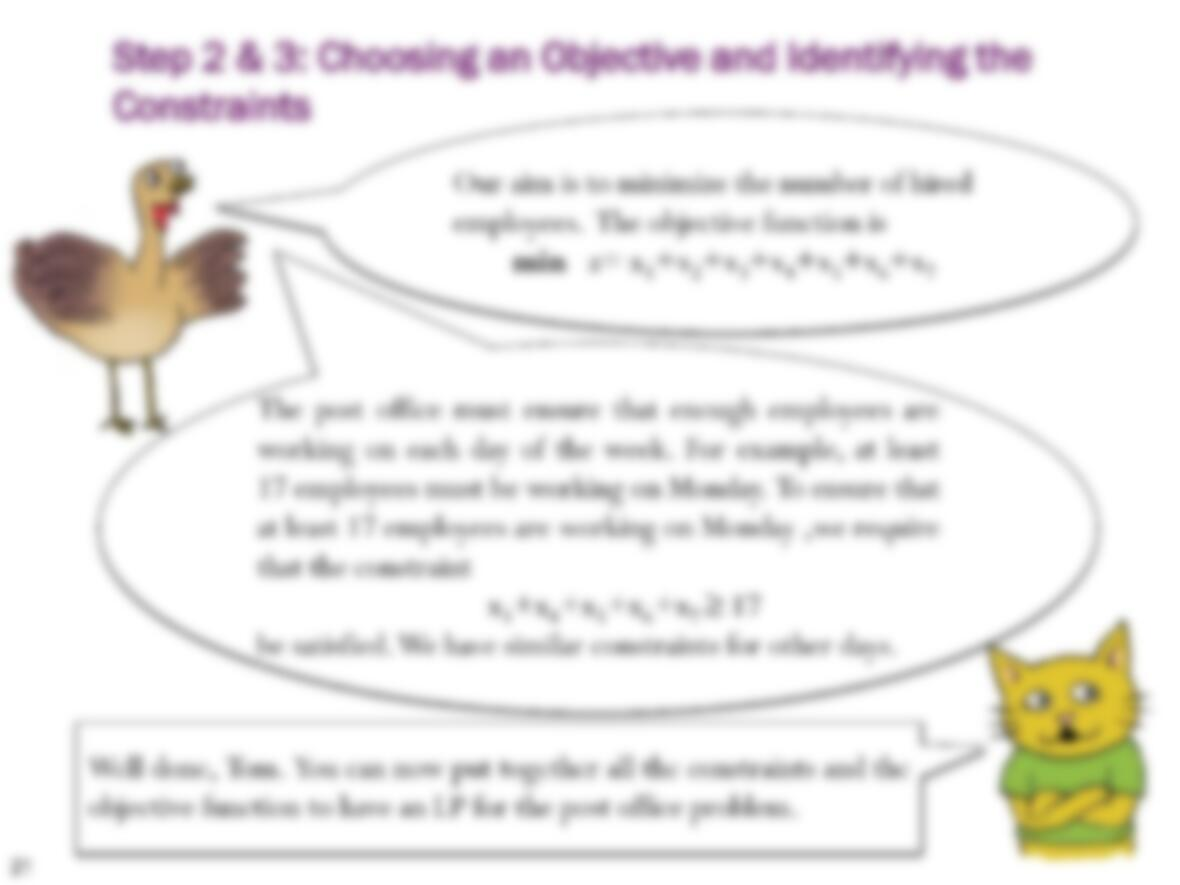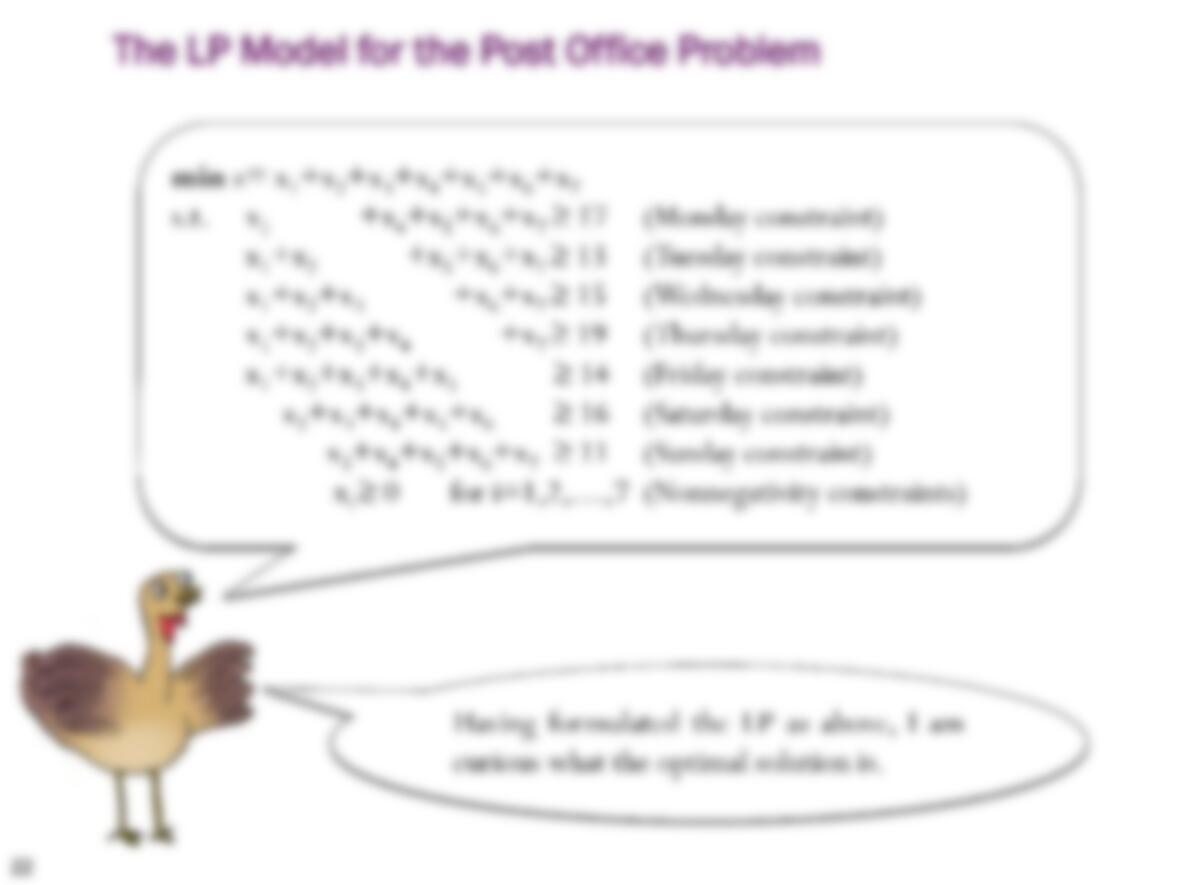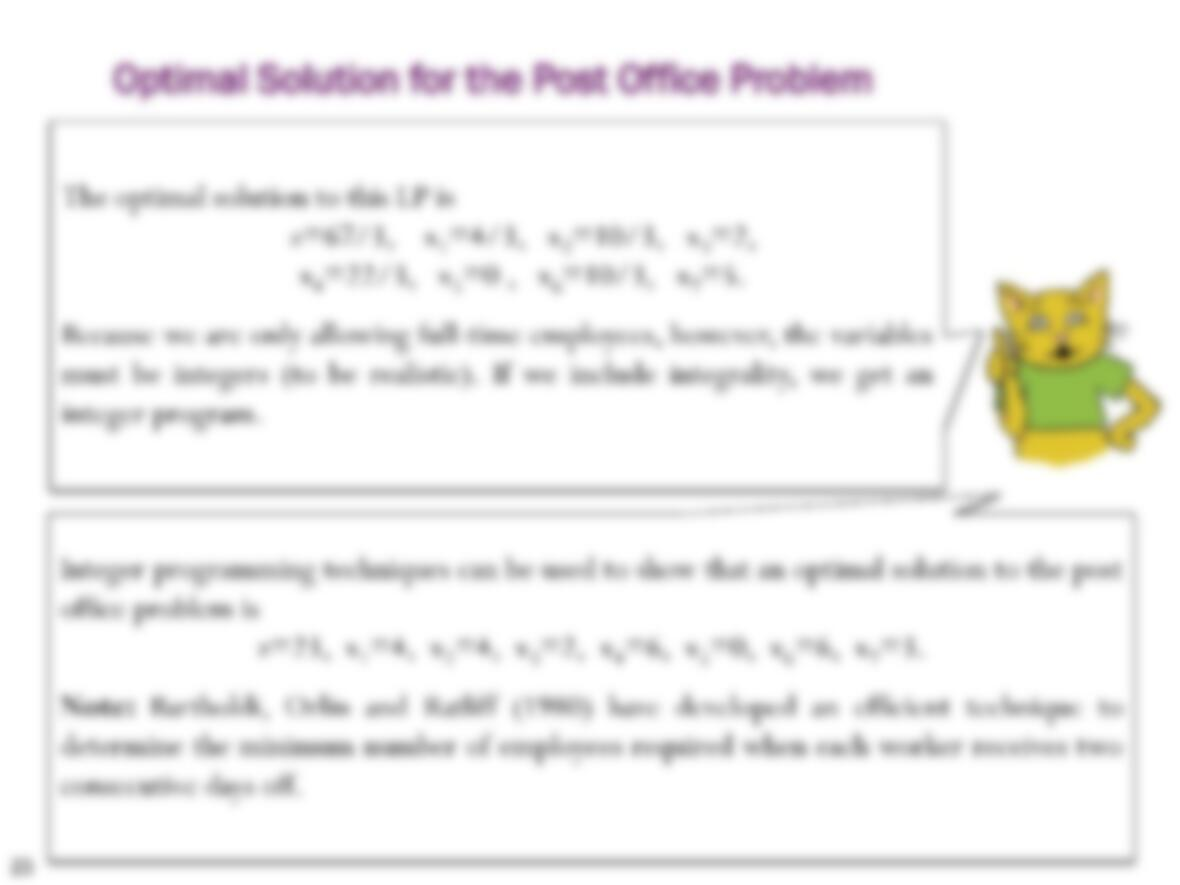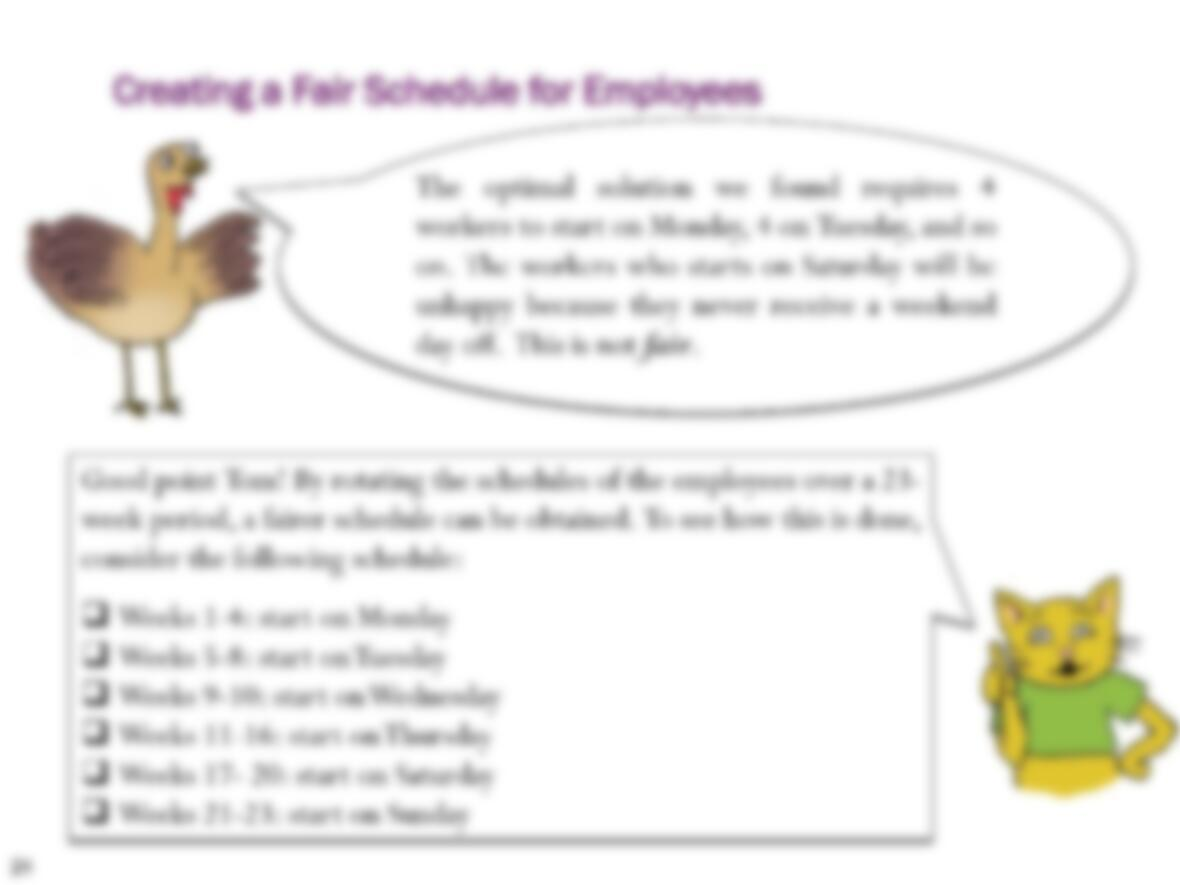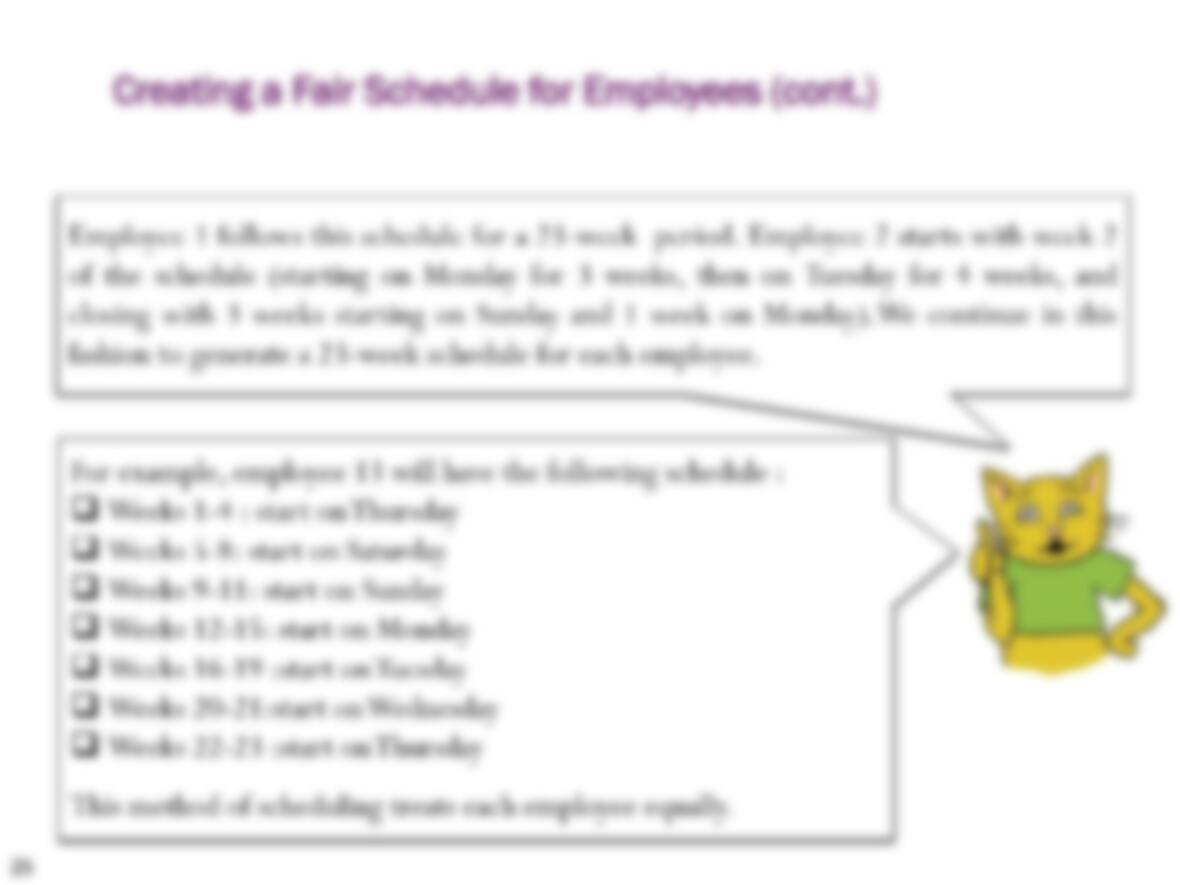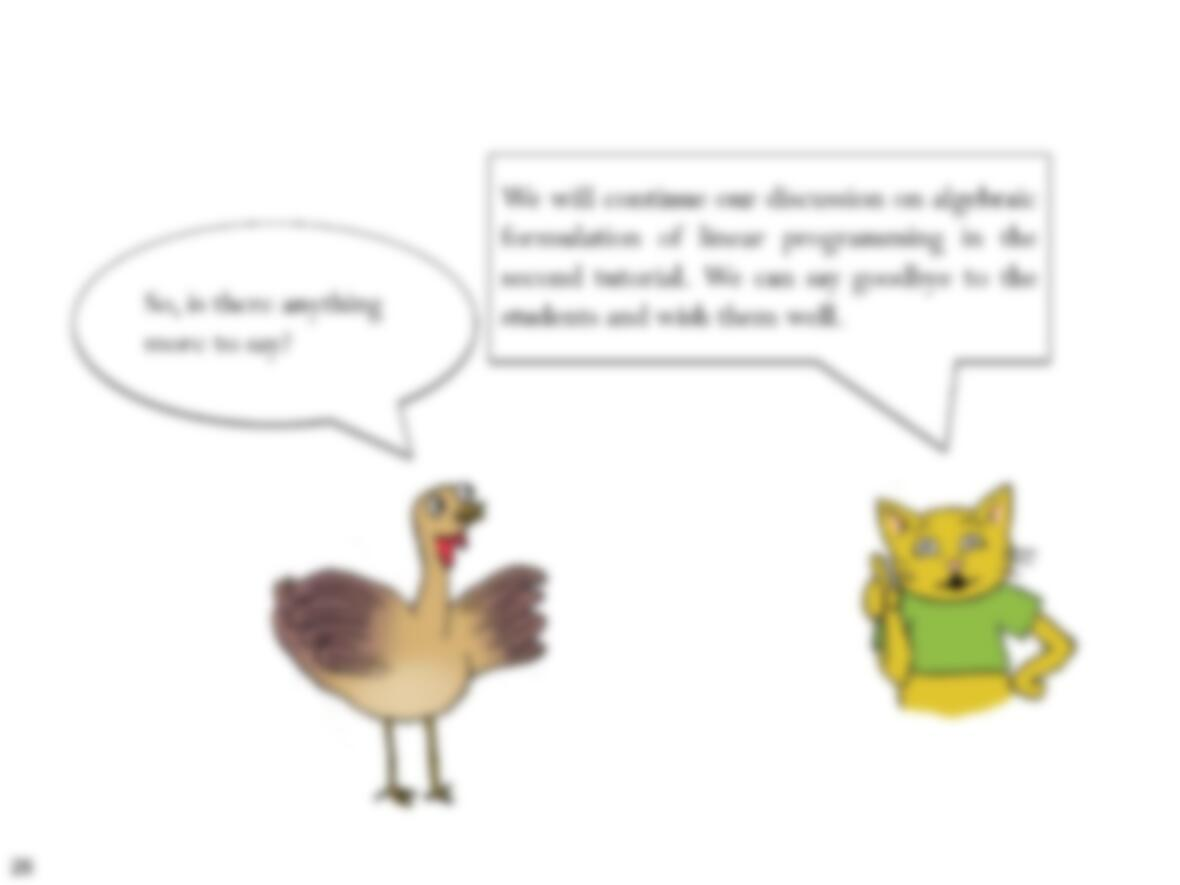Type
Essay
Pages
1 pages
Word Count
2724 words
School
Massachusetts Institute
Course Code
MIT 15.03

# Optimization

September 7, 2020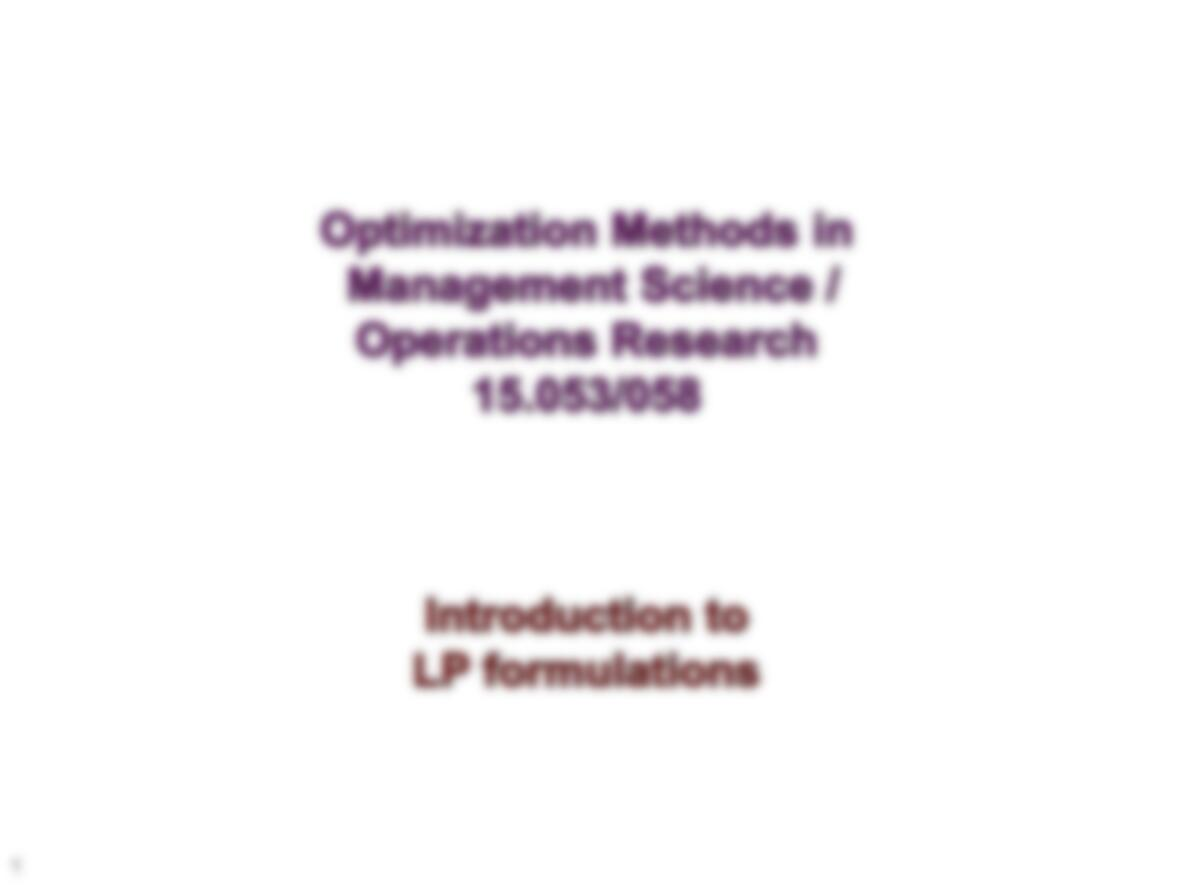1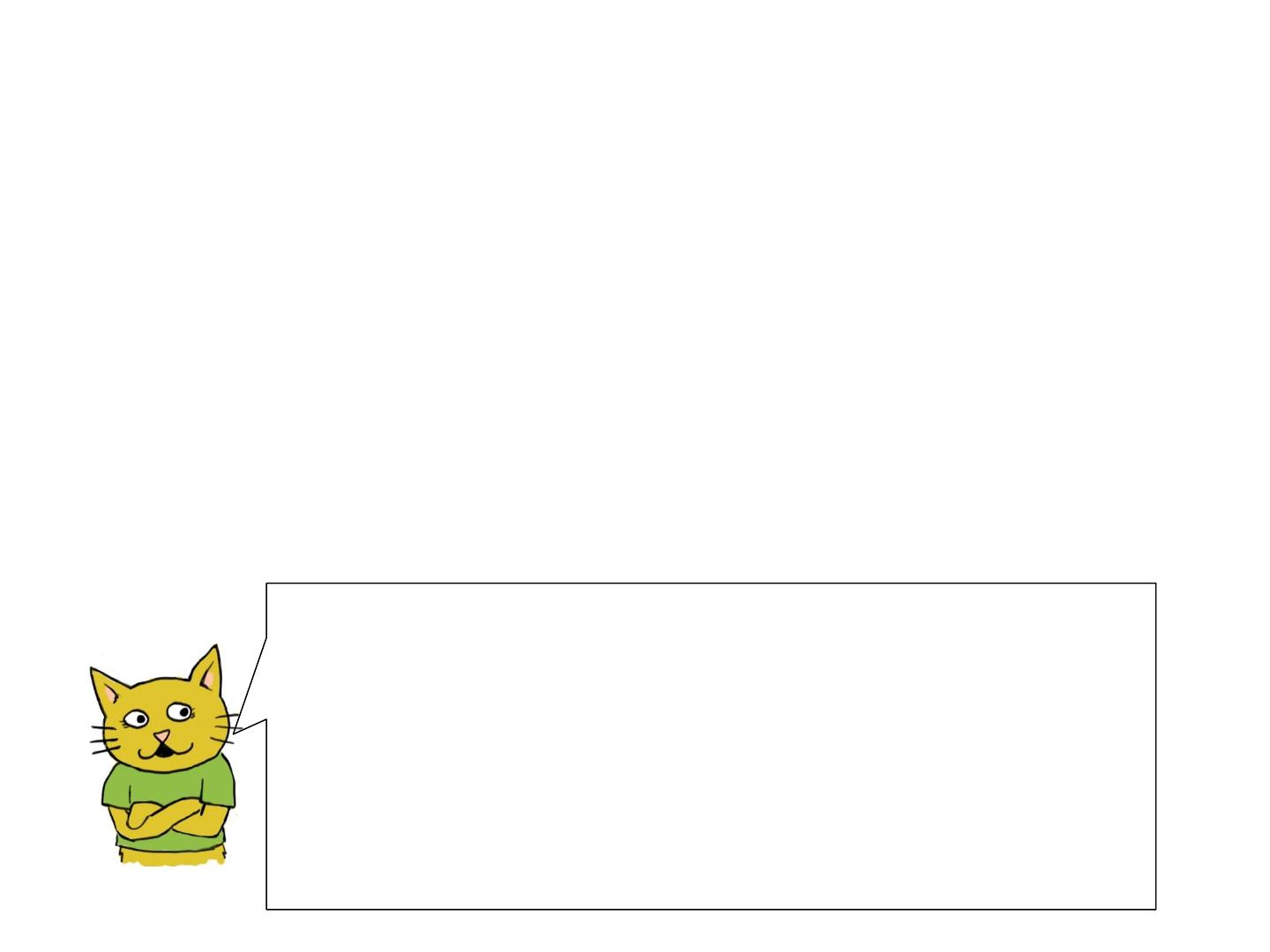2
Linear Programming
Optimization is an important and fascinating area of management science
and operations research. It helps to do less work, but gain more.
Applicability: There are many real-
world applications that can be
modeled as linear programming;
Solvability: There are theoretically
and practically efficient techniques
for solving large-scale problems.
Hi! My name is Cathy. I will guide you in tutorials during the
semester.
In this tutorial, we introduce the basic elements of an LP and
present some examples that can be modeled as an LP. In the
next tutorials, we will discuss solution techniques.
Linear programming (LP) is a
central topic in optimization. It
provides a powerful tool in
modeling many applications. LP
has attracted most of its attention
in optimization during the last six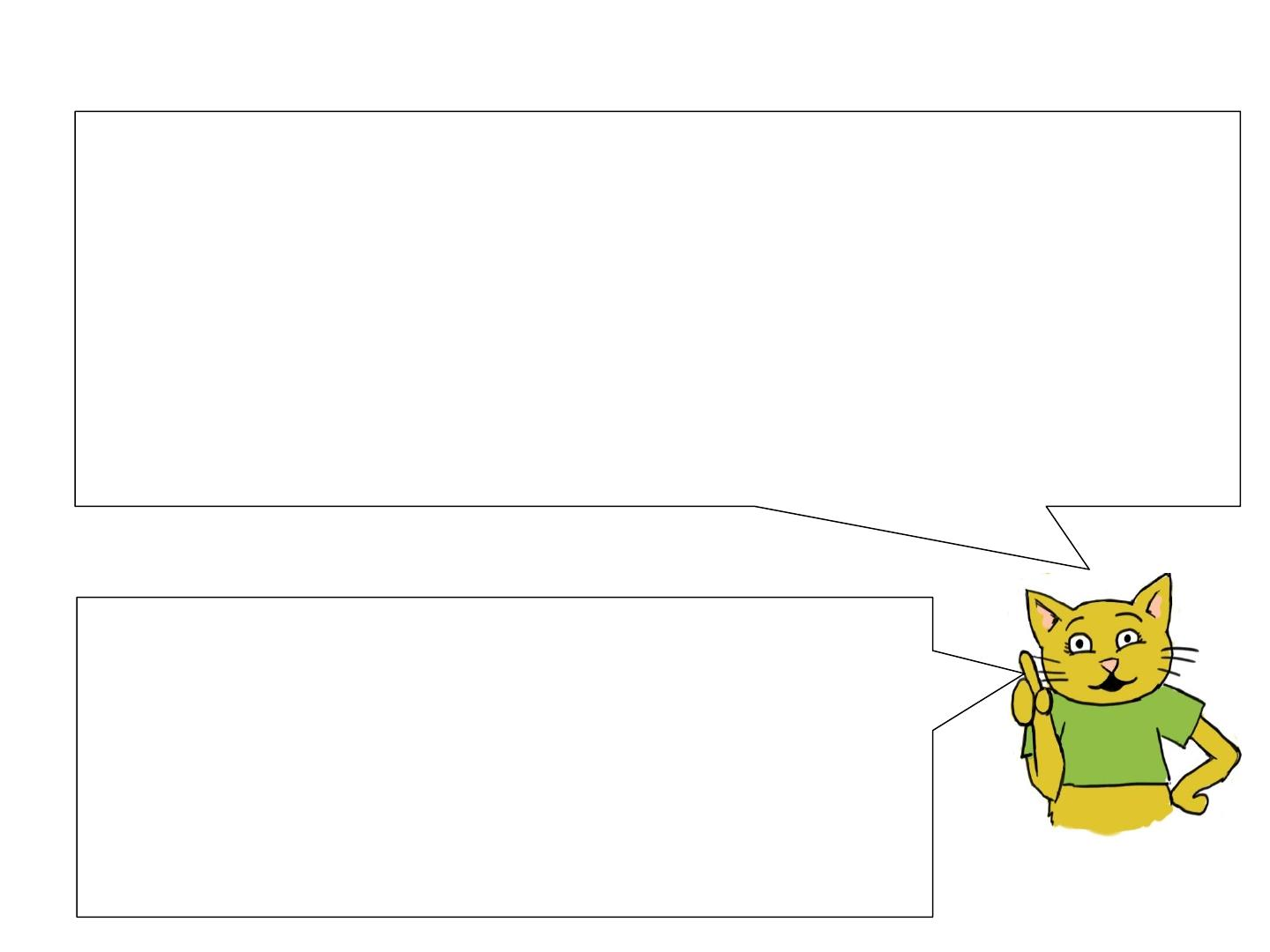3
Basic Components of an LP:
Each optimization problem consists of three elements:
decision variables: describe our choices that are under our control;
objective function: describes a criterion that we wish to minimize
(e.g., cost) or maximize (e.g., profit);
constraints: describe the limitations that restrict our choices for
decision variables.
Formally, we use the term linear programming (LP)
to refer to an optimization problem in which the objective
function is linear and each constraint is a linear inequality
or equality. I’ll discuss these features soon.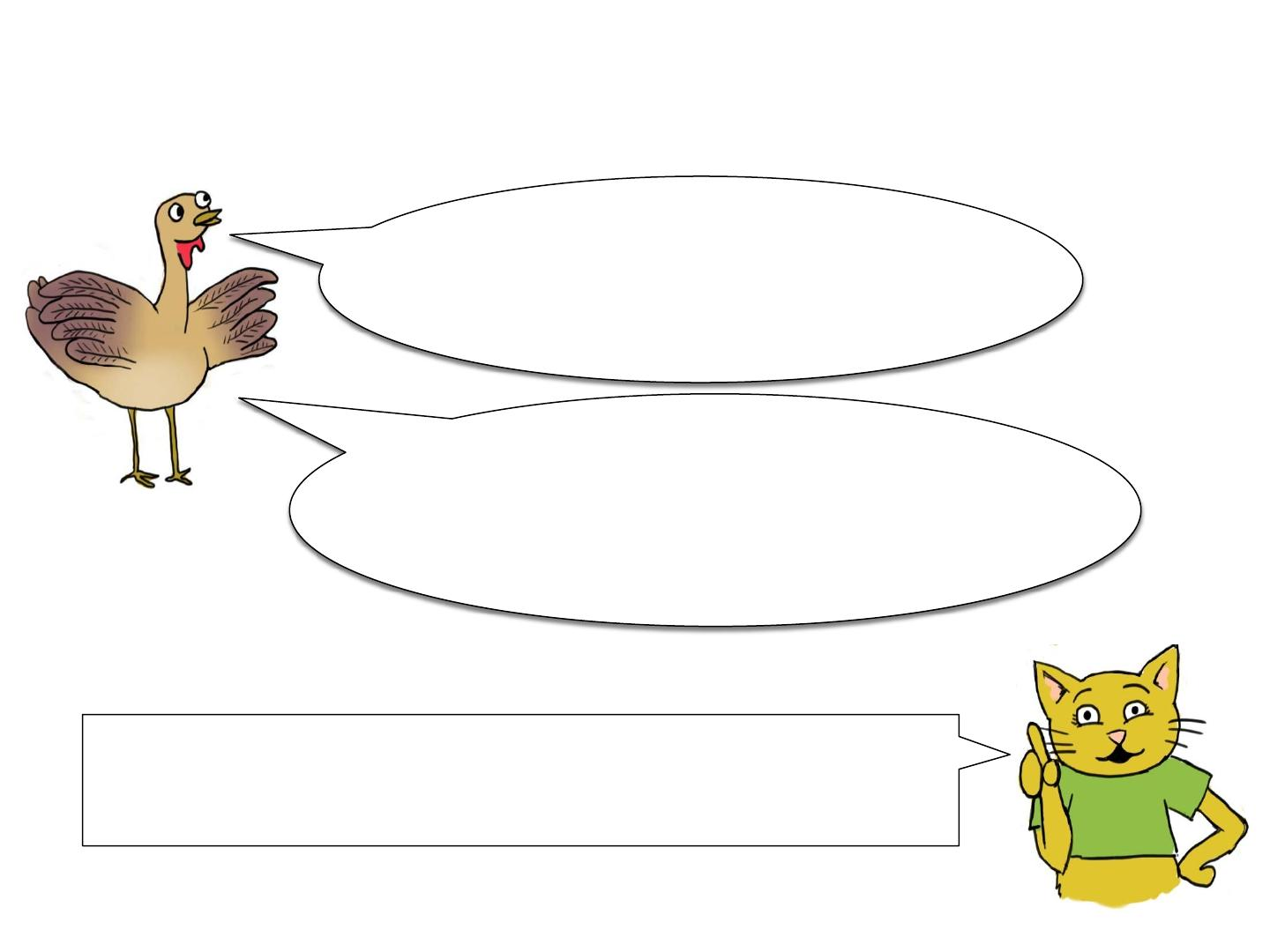4
An Introductory Example
I am a bit confused about the LP
elements. Can you give me more
details.
Let’s start with an example. I’ll describe it first in words, and then
we’ll translate it into a linear program.
Oh! I forgot to introduce myself. I am To m ; a
new member of the 15.053 class. I am
interested in learning linear programming. I
will be with you during the tutorials.An Introductory Example
Problem Statement: A company makes two products (say, P and Q)
using two machines (say, A and B). Each unit of P that is produced
requires 50 minutes processing time on machine A and 30 minutes
processing time on machine B. Each unit of Q that is produced requires
24 minutes processing time on machine A and 33 minutes processing
time on machine B.
Machine A is going to be available for 40 hours and machine B is available
for 35 hours. The profit per unit of P is \$25 and the profit per unit of Q
is \$30. Company policy is to determine the production quantity of each